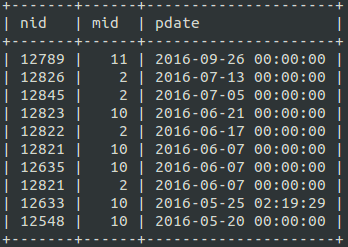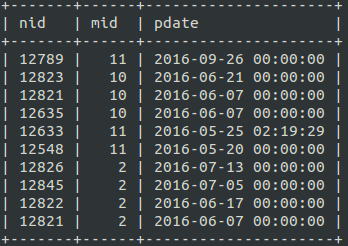6,727 Views |

We have built a website using Drupal, One of my client requirement is to show content, based on his permission. So we have used Nodeaccess (https://www.drupal.org/project/nodeaccess) module.

In Drupal has roles and mulitple roles can be assigned for each user. The real requirement was to show content of users with any roles assigned first, except Authenticate user role, then show the content of authenticate users i.e we need to first group the content with users role id greater than 2, then we need to show the content of users only has role 2 (authenticate role)

For example I have three roles id 11,10 and 2 As per the query need to GROUP BY 11,10 ORDER BY DATE, GROUP BY 2 ORDER BY DATE, tried with the union, first query will get result only rid 10 and 11, second query will get result only rid 2, here is the query

## SQL

 `(``SELECT` `n.nid, ``max``(na.gid) ``as` `mid, fav.field_date_posted_value ``as` `pdate ``FROM` ``node` ``as` `n` `  ``JOIN` `nodeaccess ``AS` `na ``ON` `na.nid = n.nid` `  ``LEFT` `JOIN` `field_data_field_date_posted ``AS` `fav ``ON` `fav.entity_id = n.nid` `  ``WHERE` `(na.gid ``IN``(10,11) ``AND` `(n.status = ``'1'``) ``AND` `(n.type ``IN`  `(``'article'``,``'blog'``)) )` `  ``GROUP` `BY` `n.nid )` `UNION` `ALL` `(``SELECT` `n.nid, ``max``(na.gid) ``as` `mid, fav.field_date_posted_value ``as` `pdate ``FROM` ``node` ``as` `n` `  ``JOIN` `nodeaccess ``AS` `na ``ON` `na.nid = n.nid` `  ``LEFT` `JOIN` `field_data_field_date_posted ``AS` `fav ``ON` `fav.entity_id = n.nid` `  ``WHERE` `(na.gid ``IN``(2) ``AND` `(n.status = ``'1'``) ``AND` `(n.type ``IN`  `(``'article'``,``'blog'``)) )` `  ``GROUP` `BY` `n.nid)` `ORDER` `BY` `pdate ``DESC` `LIMIT 10`

Result I got is roles are mixed in the entire result, this is because the union will apply order by only after populating entire result.But we need the result like thisSo after doing google search, if found a hint here at stackoverflow(http://stackoverflow.com/questions/8685168/mysql-order-by-with-union-doesnt-seem-to-work)

The hint i got from the above said website is to declare a virtual column as 1 with alias as ob and in second query again a virtual column as 2 with same alias ‘ob’ used in the first query, than at atlast use this alias ‘ob’ in the order by we will get the expected result.

I applied the hint and got the expected result

So I have Changed my query Like

 `SELECT` `n.nid, ``max``(na.gid) ``as` `mid,fav.field_date_posted_value,` `UNIX_TIMESTAMP(fav.field_date_posted_value) ``as` `pdate, 1 ``as` `ob ``FROM` ``node` ``as` `n` `  ``JOIN` `nodeaccess ``AS` `na ``ON` `na.nid = n.nid` `  ``LEFT` `JOIN` `field_data_field_date_posted ``AS` `fav ``ON` `fav.entity_id = n.nid` `  ``WHERE` `(na.gid ``IN``(10,11) ``AND` `(n.status = ``'1'``) ``AND` `(n.type ``IN`  `(``'article'``,``'blog'``)) )` `  ``GROUP` `BY` `n.nid ` ` ` ` ` `UNION` `ALL` ` ` `SELECT` `n.nid, ``max``(na.gid) ``as` `mid,fav.field_date_posted_value,` `UNIX_TIMESTAMP(fav.field_date_posted_value) ``as` `pdate, 2 ``as` `ob ``FROM` ``node` ``as` `n` `  ``JOIN` `nodeaccess ``AS` `na ``ON` `na.nid = n.nid` `  ``LEFT` `JOIN` `field_data_field_date_posted ``AS` `fav ``ON` `fav.entity_id = n.nid` `  ``WHERE` `(na.gid ``IN``(2) ``AND` `(n.status = ``'1'``) ``AND` `(n.type ``IN`  `(``'article'``,``'blog'``)) )` `  ``GROUP` `BY` `n.nid  ` ` ` `  ``ORDER` `BY` `ob ``ASC``, pdate ``DESC`

Now i got the expected resultThis method has one disadvantage i.e mysql will create a temporary table to produce this result, this might cause performance issue, in high traffic website.

Message us